# Riemann hypothesis, generalized

A statement about the non-trivial zeros of Dirichlet-functions (cf. Dirichlet-function), Dedekind zeta-functions (cf. Zeta-function) and several other similar functions, similar to the Riemann hypothesis (cf. Riemann hypotheses) on the non-trivial zeros of the Riemann zeta-function. In the case of Dirichlet zeta-functions the generalized Riemann hypothesis is called the extended Riemann hypothesis.

For Dirichlet-functions it is not even known whether there exist real zeros in the interval(Siegel zeros). This is important in connection with the class number of quadratic fields (see also Quadratic field; Siegel theorem).
Letbe an algebraic number field,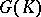the group of fractional ideals ofand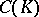its idèle class group (cf. Idèle; Fractional ideal). Letbe a quasi-character on, i.e. a continuous homomorphism ofinto the group of non-zero complex numbers. Then for an idèleone has, where for each,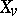is a quasi-character of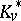which is equal to unity on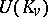, the units of the local completion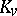, for almost-all. Letbe a finite subset of the valuations onincluding the Archimedian ones,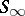. A functioncan now be defined onby setting for all prime ideals,and extendingmultiplicatively. These functions are called Hecke characters or Grössencharakters. Given such a character, the Hecke zeta-function ofis defined by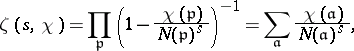whereis the absolute norm. The functionis also called-series, Dirichlet-series (whenis a Dirichlet character) or Hecke-function with Grössencharakter; it is also denoted by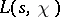. Ifone obtains the Dedekind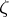-function. For Dirichlet-series the generalized Riemann hypothesis states that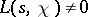if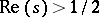.